Function Repository Resource:

# FormatDataset

Format a dataset using a given set of option values

Contributed by: Seth J. Chandler
 ResourceFunction["FormatDataset"][rule1,…] represents an operator that applies the formatting options rulei to a Dataset.

## Details

The arguments can be any options accepted by Dataset.

## Examples

### Basic Examples (2)

Apply formatting rules to a Dataset:

 In:=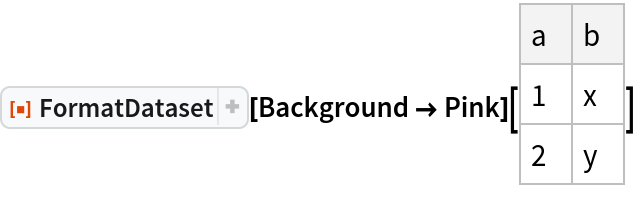Out=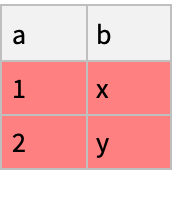Create a Dataset:

 In:=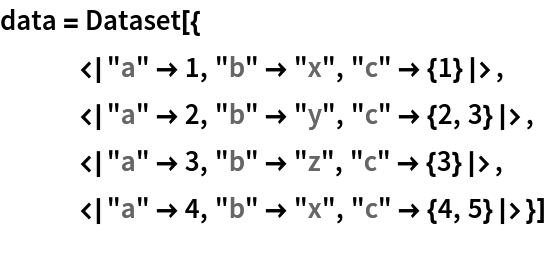Out=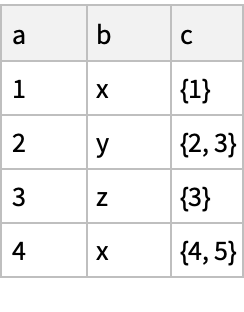Reformat it so that only three rows show and the background is cyan:

 In:=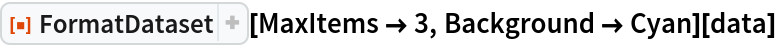Out=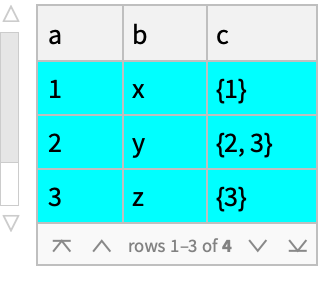Apply a Query to data and format the results using the "Web" theme:

 In:=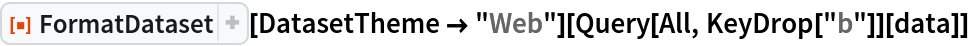Out=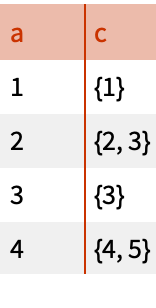Apply a Query and use a postfix form of the function with iconized arguments to format the results using multiple styling options:

 In:=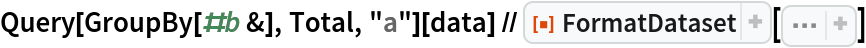Out=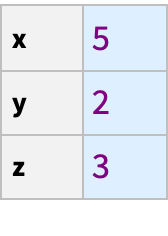### Scope (1)

If the data to which the function is applied is not a Dataset to begin with, the function will convert it into a formatted Dataset:

 In:=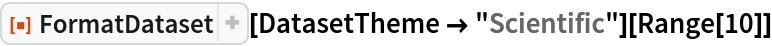Out=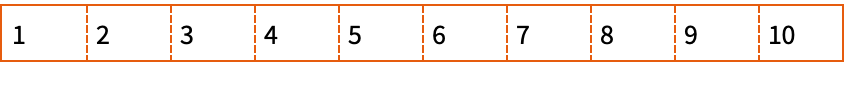### Applications (3)

Take data on executions in the United States:

 In:=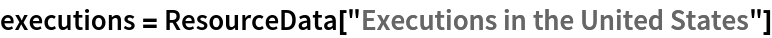Out=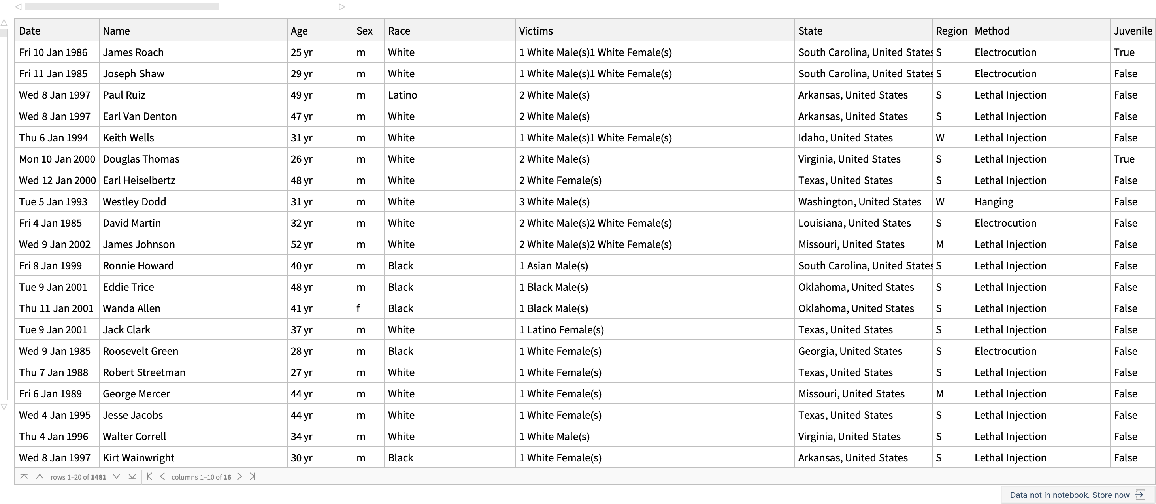Group the data by state, find the distribution of executed persons by race, reverse sort the states by total number of executions:

 In:=Display the results of the query by showing just the 5 states with the highest number of executions, while using a "Monochrome" theme:

 In:=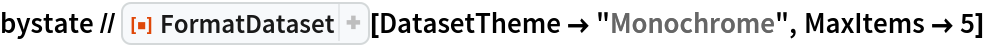Out=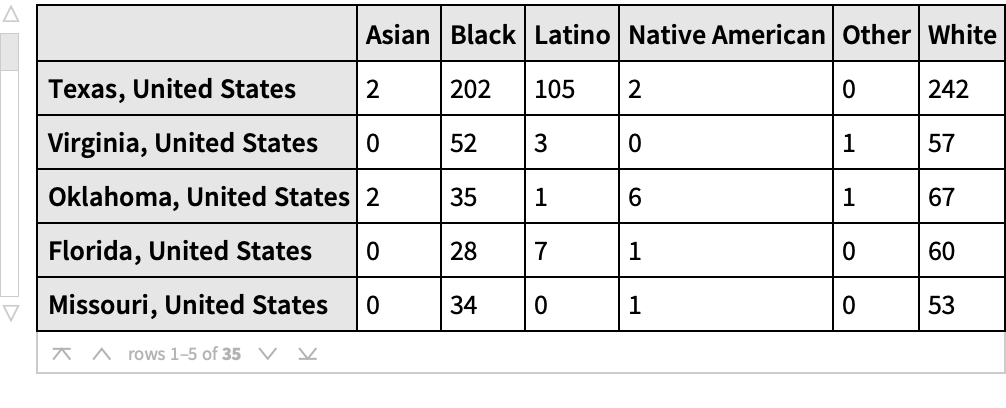Highlight in lighter purple the number of Black persons executed:

 In:=Out=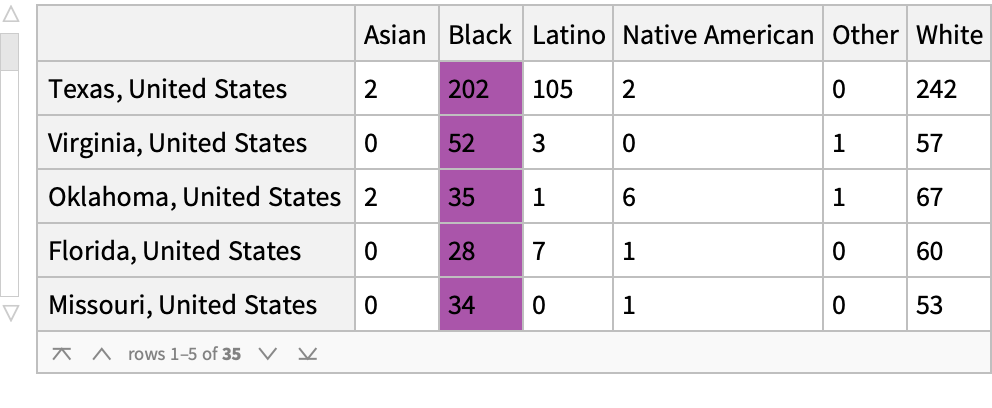One can store a format as a named variable and then deploy it on different datasets:

 In:=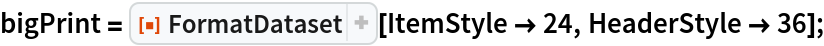In:=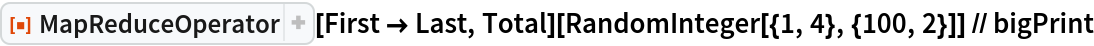Out=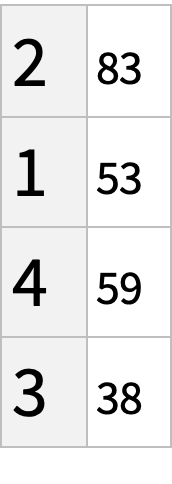In:=Out=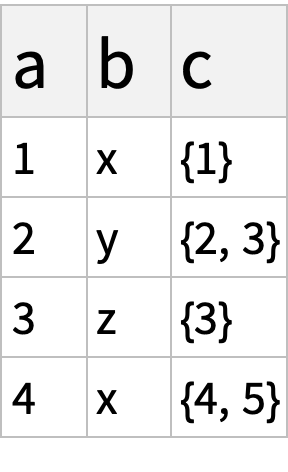One can create a function using FormatDataset that permits customization and experimentation with formats:

 In:=In:=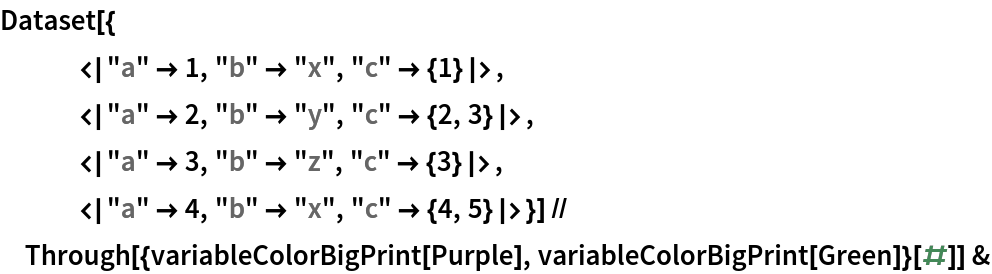Out=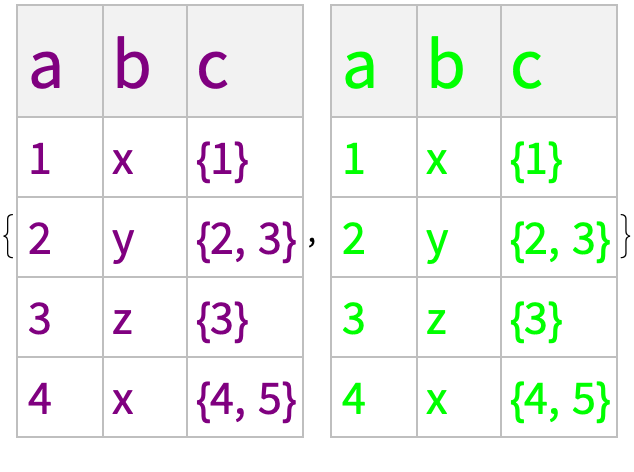### Properties and Relations (1)

The function compliments the resource function DatasetWithHeaders by permitting the results to be formatted:

 In:=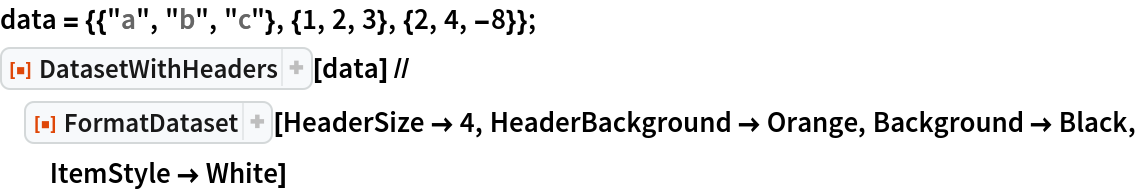Out=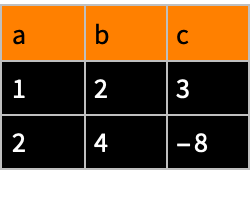Seth J. Chandler

## Version History

• 1.0.0 – 29 June 2021